Courses

# Olympiad Test: Classification - 2

## 20 Questions MCQ Test | Olympiad Test: Classification - 2

Description
This mock test of Olympiad Test: Classification - 2 for Class 5 helps you for every Class 5 entrance exam. This contains 20 Multiple Choice Questions for Class 5 Olympiad Test: Classification - 2 (mcq) to study with solutions a complete question bank. The solved questions answers in this Olympiad Test: Classification - 2 quiz give you a good mix of easy questions and tough questions. Class 5 students definitely take this Olympiad Test: Classification - 2 exercise for a better result in the exam. You can find other Olympiad Test: Classification - 2 extra questions, long questions & short questions for Class 5 on EduRev as well by searching above.
QUESTION: 1

### Three of the following four are alike in a certain way so form a group select the one which does not belong to the group.

Solution: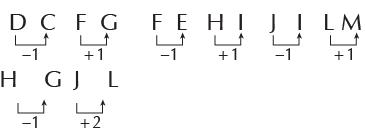QUESTION: 2

### Three of the following four are alike in a certain way so form a group select the one which does not belong to the group.

Solution: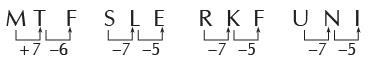QUESTION: 3

### Three of the following four are alike in a certain way so form a group select the one which does not belong to the group.

Solution: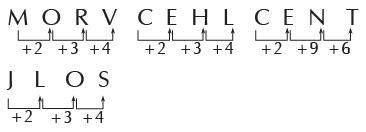QUESTION: 4

Three of the following four are alike in a certain way so form agroup select the one which does not belong to the group.

Solution: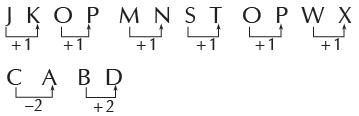QUESTION: 5

Three of the following four are alike in a certain way so form a group select the one which does not belong to the group.

Solution: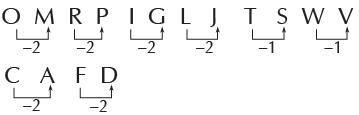QUESTION: 6

Three of the following four are alike in a certain way so form a group select the one which does not belong to the group.

Solution: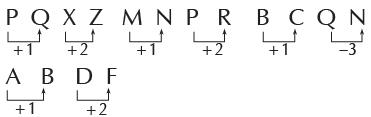QUESTION: 7

Three of the following four are alike in a certain way so form a group select the one which does not belong to the group.

Solution: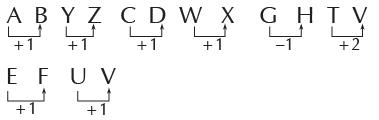QUESTION: 8

Three of the following four are alike in a certain way so form a group select the one which does not belong to the group.

Solution: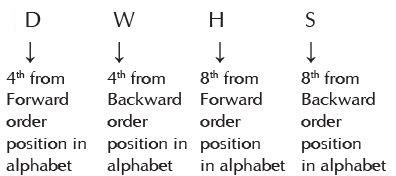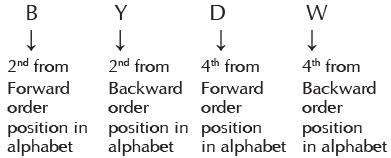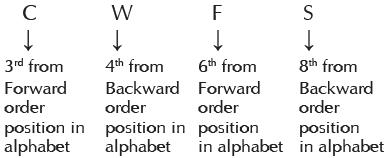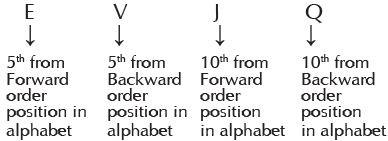QUESTION: 9

Three of the following four are alike in a certain way so form a group select the one which does not belong to the group.

Solution: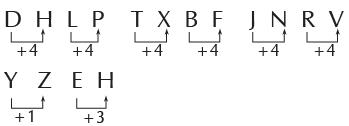QUESTION: 10

Three of the following four are alike in a certain way so form a group select the one which does not belong to the group.

Solution: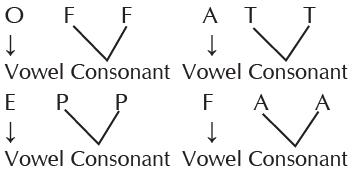QUESTION: 11

Three of the following four are alike in a certain way so form a group select the one which does not belong to the group.

Solution: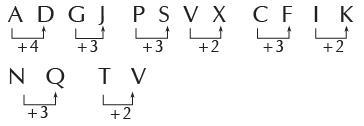QUESTION: 12

Three of the following four options are alike in a certain way andform a group. Select the one which does not belong to the group.

Solution:

Except (D), all other numbers are
square of natural numbers. 64 = 82,
196 = 142, 900 = 302.

QUESTION: 13

Three of the following four options are alike in a certain way and form a group. Select the one which does not belong to the group.

Solution:

Except (D), in all other options, second number is double of the first number.

QUESTION: 14

Three of the following four options are alike in a certain way and form a group. Which is the one that does not belong to the group?

Solution:

Except (A), all other numbers are
cubes of natural numbers.

QUESTION: 15

Three of the following four options are alike in a certain way and form a group. Select the one which does not belong to the group.

Solution:

Except (D), in all other numbers, second number is obtained by reversing the digits of first number.

QUESTION: 16

Three of the following four options are alike in a certain way and form a group. Select the one which does not belong to the group.

Solution:

Except (B), in other numbers, difference between second number and first number is 10.

QUESTION: 17

Three of the following four options are alike in a certain way and form a group. Select the one which does not belong to the group.

Solution:

Except (B), all other numbers are divisible numbers.

QUESTION: 18

Three of the following four options are alike in a certain way and form a group. Select the one which does not belong to the group.

Solution:

Except (A), all other numbers are
divisible by 9.

QUESTION: 19

Three of the following four options are alike in a certain way and form a group. Select the one which does not belong to the group.

Solution:

Except (C), all letters have number corresponding to the position in the alphabet.

QUESTION: 20

Three of the following four options are alike in a certain way and form a group. Select the one which does not belong to the group.

Solution:

Except (D), all numbers are divisible by 4.